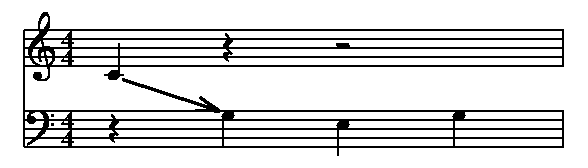## Converting a value to a string

A macro name can be placed inside ` ` to get a string version of it. That effectively puts double quotes around the macro value, and does any necessary escaping. That may be useful, for example, if you have a numeric value that you want to print, or want to use something both literally and as a string, as in this example:

```// Choose a clef
ifdef USE_BASS
define CLEF bass@
else
define CLEF treble@
endif

score
// Use CLEF literally
clef=CLEF
music
// Use CLEF as a string
rom above 1: 1 "This uses the " + `CLEF` + " clef";
1: c;d;e;c;
bar
```

There is also a string() function available, which maps a number to a string, using a transform. Currently, there are four transforms available. A transform of "LET" maps the number to one or two upper case letters, while "let" maps to lower case letters. Similarly, "ROM" and "rom" will map to upper and lower case Roman numerals, respectively. Some examples:
 Input Result string(1, "LET") "A" string(2, "LET") "B" string(26, "let") "z" string(27, "let") "aa" string(3, "ROM") "III" string(36, "rom") "xxxvi"

Those might be useful for things like labeling movements or songs in a collection, if you wanted to use letters or Roman numerals rather than numbers. To demonstrate the functionality, here is how an outline could be created:

```eval L1 = 1 @
eval L2 = 1 @
eval L3 = 1 @
eval L4 = 1 @

define LEVEL1(TEXT)
left nl string(L1, "ROM") + ". " + `TEXT`
eval L1 = L1+1 \@
eval L2 = 1 \@
eval L3 = 1 \@
eval L4 = 1 \@
@

define LEVEL2(TEXT)
left nl "    " + string(L2, "LET") + ". " + `TEXT`
eval L2 = L2+1 \@
eval L3 = 1 \@
eval L4 = 1 \@
@

define LEVEL3(TEXT)
left nl "        " + string(L3, "rom") + ". " + `TEXT`
eval L3 = L3+1 \@
eval L4 = 1 \@
@

define LEVEL4(TEXT)
left nl "            " + string(L4, "let") + ". " + `TEXT`
eval L4 = L4+1 \@
@
block

LEVEL1(This is the first top level item)
LEVEL2(This is a second level item)
LEVEL2(This is another second level item)
LEVEL3(This is a third level item)
LEVEL4(This is a fourth level item)
LEVEL3(This is another third level item)
LEVEL4(A new fourth level item)
LEVEL1(This is another top level item)
LEVEL2(This is a new second level item)
```The range for LET and let is 1 to 702 (A, B, C, ... Y, Z, AA, AB, AC, ... ZZ), and the range for ROM and rom is 1 to 3999.